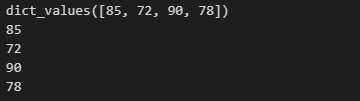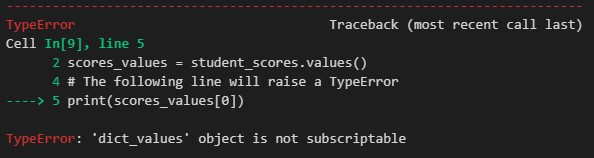## Python ‘dict_values’ object is not subscriptable

##### Aniekan

While working with Python dictionaries, we might encounter the following error: `"TypeError: 'dict_values' object is not subscriptable."`. If you’ve faced this error or want to ensure you never stumble upon it, you’ve come to the right place.

In this article, we will discuss the `dict_values`object and then uncover why the programmer could encounter the error. By the end of this article, you will gain a better understanding of why the error occurs, and how to avoid running into it. So, let us start our exploration.

## The `dict_values` Object

A Python dictionary stores data as key-value pairs. In order to access all values in a dictionary at once, the dictionary object provides the `values()` method. The `values()` method, when called, returns a `dict_values` object that represents all the values contained within the dictionary.

The `dict_values` object is iterable that provides access to all values in the dictionary. Also, any changes in the dictionary, like adding a new key-value pair, will be reflected in the `dict_values` object. The `dict_values` object provides a way to access the dictionary’s values without the need for the associated keys.

The code example below defines a dictionary object and accesses the `dict_values` object.

``````# Create a dictionary containing student scores
student_scores = {'Alice': 85, 'Bob': 72, 'Charlie': 90, 'David': 78}

# Get the 'dict_values' object using the .values() method
scores_values = student_scores.values()

# Output the 'dict_values' object
print(scores_values)  # Output: dict_values([85, 72, 90, 78])

# Loop through the 'dict_values' object
for score in scores_values:
print(score)  # Output: 85, 72, 90, 78
``````

The output is shown below:As can be seen from the output, the first call to `print`returns the `dict_values` iterable. Within the `for` loop, however, the `dict_values`object is iterated over so as to allow access to individual values in the iterable. As each value is accessed, we print it to the console.

The next code example demonstrated the dynamic nature of the `dict_values` object.

``````# Adding a new key-value pair to the dictionary
student_scores['Eve'] = 95

# Output the 'dict_values' object after adding a new entry
print(scores_values)  # Output: dict_values([85, 72, 90, 78, 95])

# Removing a key-value pair from the dictionary
del student_scores['Charlie']

# Output the 'dict_values' object after removing an entry
print(scores_values)  # Output: dict_values([85, 72, 78, 95])
``````

The first change made to the dictionary was to add the new key-value pair `"Eve": 95`. The `dict_values` object referenced by the `score_values` variable is updated to reflect this change (as seen in the output within the comment).

The second change made was to delete the key-value pair `"Charlie":  90`. The output also show that the delete change was also reflected in the `dict_values` output.

However, unlike lists, or tuples, the `dict_values` object does not support indexing or slicing. This is because it is a dynamic view of the dictionary’s current state.

## Subscriptable Objects in Python

Subscriptable objects allow us to make use of square brackets `[]` to access individual elements within the object.  With this feature, we can access items by index/position, or by key.

An example of a subscriptable object is a list. Items in a list can be accessed by index by utilizing the `[]` notation and including the position/index of the object in between the square brackets. See the code example below:

``````my_list = [10, 20, 30, 40]
print(my_list)  # Output: 10
``````

While a list is subscriptable (also tuples, and strings), any object of the type `dict_values` is not subscriptable, and thus, does not allow direct access to the elements within them. See the code example below of an attempt to access elements in a `dict_value` object by subscripting:

``````student_scores = {'Alice': 85, 'Bob': 72, 'Charlie': 90}
scores_values = student_scores.values()

# The following line will raise a TypeError
print(scores_values)
``````

The output we get is shown below:As seen from the error message, we cannot directly access individual items inside the `dict_values` object by making use of indexing/subscripting.

## Some Solutions to this Error

In order to circumvent this error that arises when we try to access a `dict_values` object by indexing, we need to understand that a `dict_values` object is not subscriptable like a list, or string. Instead of trying to directly access the items in a `dict_values` object, we can make use of alternative approaches. Some of them will be discussed in the subsections that follow.

### Iterating over `dict_values`

Since the `dict_values` object is iterable, we can use the `for` loop to iterate over each dictionary value it references. Let us see this in action in the code snippet below:

``````student_scores = {'Alice': 85, 'Bob': 72, 'Charlie': 90}
scores_values = student_scores.values()

# Using a for loop to iterate over 'dict_values'
for score in scores_values:
print(score)
``````

This approach successfully accesses each value referenced by the `dict_value` object as seen in the output below.### Converting `dict_values` to other subscriptable objects

We might have a specific use case that requires that we make use of indexing. If this situation arises, we will need to convert the `dict_values` object into a subscriptable object, like a list, or tuple. Then we use the `[]` notation to access the values individually. See an example in the code snippet below.

``````student_scores = {'Alice': 85, 'Bob': 72, 'Charlie': 90}
scores_values = student_scores.values()

# Convert 'dict_values' to a list
scores_list = list(scores_values)

# Accessing elements using index
print(scores_list)  # Output: 72
``````

We have to note, however, that this conversion will create a separate copy of the dictionary values in the list. As a result, any changes to the underlying dictionary will not be reflected in the newly created list of dictionary values.

### Utilizing dictionary methods to access elements

There are several built-in methods provided by Python dictionaries to access the contents of a dictionary. One of them is the `get()` method. the example below illustrates how to make use of the `get()` method to access values within a dictionary.

``````student_scores = {'Alice': 85, 'Bob': 72, 'Charlie': 90}

# Using .get() method to retrieve a specific value
alice_score = student_scores.get('Alice')
print(alice_score)  # Output: 85``````

The code makes use of the `get()` method to directly access the value associated with the dictionary key `'Alice'`. The attempt successfully retrieves that value `85` without the error occurring.

To conclude, in order to avoid running into the error `'TypeError: 'dict_values' object is not subscriptable'`, we need to appreciate that the `dict_values` object in Python is iterable that does allow indexing. However, there are ways we could circumvent this error, which we have covered in this article.

If you liked this article, you are welcome to explore our knowledgebase of Python articles that can assist you in learning more about Python and becoming a more proficient developer. In the meantime, Happy coding!